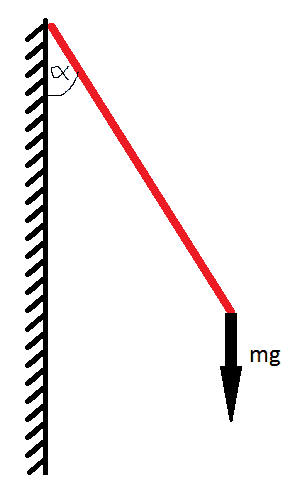# Angular velocity of a rod rotating around a vertical axis

• alaix
In summary, the conversation discusses how to find the angular velocity needed for a homogeneous rod to rotate around a vertical axis at an angle of theta with the vertical. The equation to calculate this angular velocity is given as \omega = \sqrt{3g/2L cos(θ)}. However, there is some ambiguity in the equation and it is necessary to include extra parentheses to clarify the numerator and denominator. Additionally, there is a mistake in stating that the angular acceleration is equal to d\omega/dθ, when it is actually equal to d\omega/dt.

## Homework Statement

An homogeneous rod is fixed to an extremity and is rotating around a vertical axis, doing an angle of theta with the vertical. (in the scheme I made I wrote alpha but it's theta! (θ))

If the length of the rod is L, show that the angular velocity needed to make it turn is

$\omega$ = $\sqrt{3g/2L cos(θ)}$## Homework Equations

$\tau$ = r x F
I = 1/2 m L²

$\tau$ = I$\alpha$

## The Attempt at a Solution

Here is what I tried

I considered that all the exterior forces (ie. gravity) was acting on the center of mass of the rod, which is situated in the middle, at L/2.

Therefore

Torque = r x F = 1/2 L mg sin(θ)

Torque = I$\alpha$

Where I = 1/3 mL²

Therefore

$\alpha$ = $\frac{3mgL sin(θ)}{2mL^{2}}$ = $\frac{3g sin(θ)}{2L}$

Since I'm looking for the angular VELOCITY, and since angular acceleration = d$\omega$/dθ

$\alpha$ dθ = d$\omega$

By integrating both sides I find

$\omega = -\frac{3g cos(θ)}{2L}$

Which is ALMOST the answer I'm looking for... what am I missing?

Thanks!

alaix said:

## Homework Statement

An homogeneous rod is fixed to an extremity and is rotating around a vertical axis, doing an angle of theta with the vertical. (in the scheme I made I wrote alpha but it's theta! (θ))

If the length of the rod is L, show that the angular velocity needed to make it turn is

$\omega$ = $\sqrt{3g/2L cos(θ)}$

There is a lot of ambiguity here as to what the final answer is supposed to be. You should include some extra parentheses under the square root sign to make it clear what's in the numerator and what's in the denominator.

alaix said:

## Homework Equations

Since I'm looking for the angular VELOCITY, and since angular acceleration = d$\omega$/dθ

$\alpha$ dθ = d$\omega$

No, actually that's wrong: $\alpha \neq d\omega/d\theta$. Rather, $\alpha = d\omega / dt$.